# How to Write Fractions as a Ratio

How to Write Fractions as a Ratio• The of a fraction tells us the total number of parts.
• If the denominators of our fractions are the same then we can read the of each amount using the of the fraction.
• We write the two numerators as a ratio by separating them with a colon ‘:’ .
• Here there are 3 blue counters to every 2 purple counters.

When the denominators of a fraction are the same, we can write our ratio using the numerators separated by a colon ‘:’ .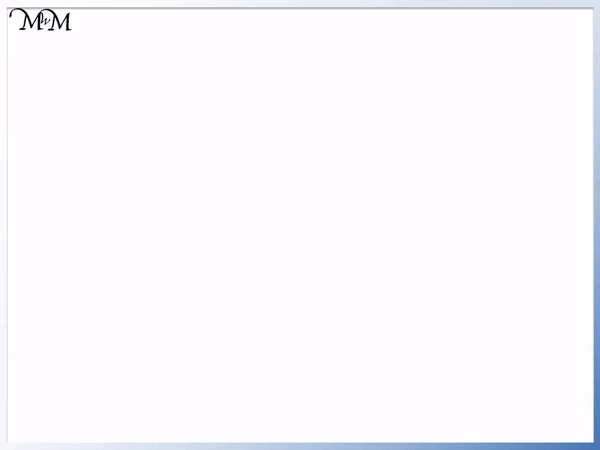• The are the same so we can simply write the using the .
• There are 11 counters in total.
• 5 are blue and 6 are purple.
• The ratio of blue counters to purple counters is 5:6.Supporting Lessons# How to Convert Fractions into a Ratio

## How to Write Fractions as a Ratio

To write fractions as a ratio, first write the fractions as equivalent fractions that have the same denominator. Then write the numerators of the fractions, each separated by a colon.

Here is an example of converting fractions into a ratio.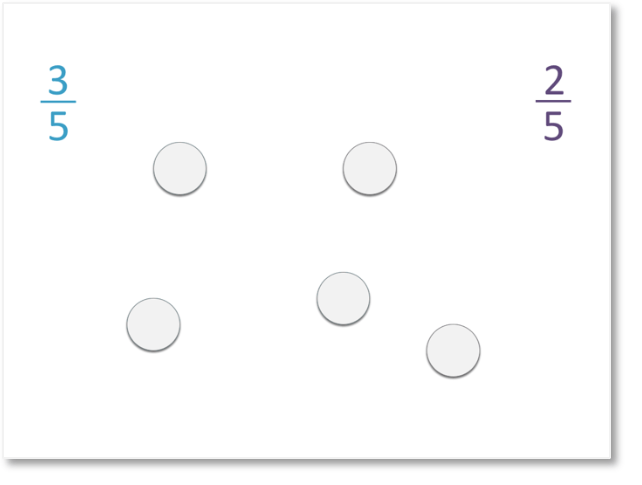Of the following 5 counters,  3 / 5   are blue and   2 / 5   are purple.

The

of 5 mean that there are 5 counters in total.

The

of 3 in   3 / 5   means that three of the counters are blue.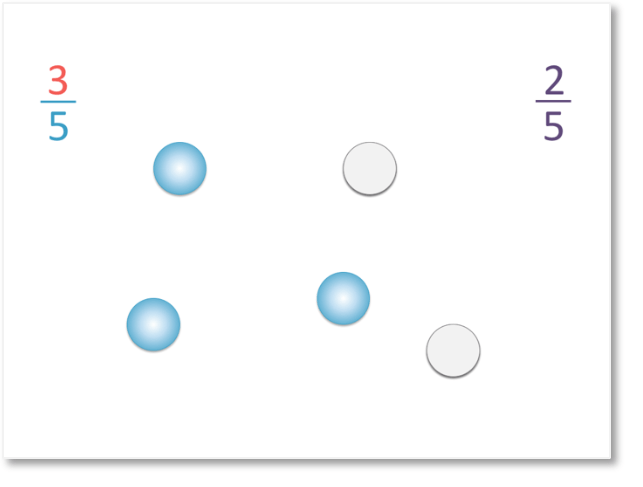The numerator of 2 in   2 / 5   means that two of the counters are purple.To write this as a ratio, we write the number of blue counters and number of purple counters.

There are three blue counters and two purple counters.

We separate the numbers in a ratio with a colon.

So, the ratio is written as 3:2.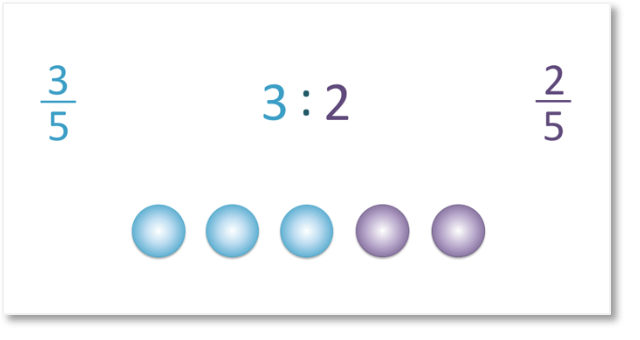We can see that there is a simple way to write the two fractions as a ratio, by comparing the numerators.Since the fractions are both out of 5, we can simply look at the numerators.

The numerator of 3 tells us that there are three blue counters, so we can write 3 in the ratio.

The numerator of 2 tells us that there are two purple counters, so we can write 2 in the ratio.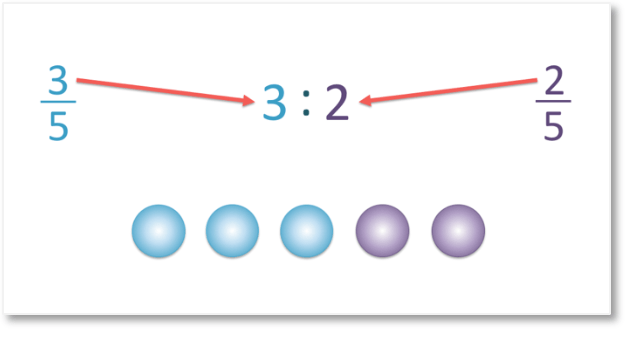Here is another example of writing two fractions as a ratio.

We have the fractions of   2 / 7   and   5 / 7  .Since the denominators are both 7, this tells us that there are 7 parts in total.

We can simply write the numerators as a ratio.

We have 2 : 5.

There is a simple check to see if the fractions have been converted to a ratio correctly. Add the numbers in the ratio to see if they add up to the denominator of the fraction.

For example, 2 + 5 = 7, which is the denominator of both fractions in the question.

Here is another example of writing fractions in a ratio.

We have   7 / 11   who are boys and   4 / 11   who are girls.

The fractions have the same denominator, so it is easy to write them as a ratio.We write the 7 and 4 as a ratio, separated by a colon.

We have the ratio 7 : 4. In this example, this means that there are 7 boys to every 4 girls.

Here is an example of comparing the fractions of the amounts of cats and dogs owned by a group of people.

We have   9 / 23   cats and   14 / 23  dogs.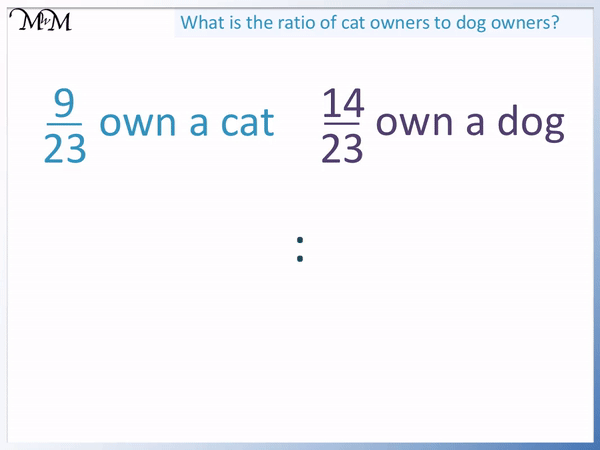Since the denominators of the fraction are the same, we can simply write the numerators in a ratio.

We have 9 : 14, which means that for every 9 cats there are 14 dogs.

## How to Convert Fractions to Ratios when the Denominators are Different

If the denominators of the fractions are not the same, then we need to use equivalent fractions to make them have a common denominator before we can write them as a ratio.

Here is an example with more than one fraction with different denominators.

We have   2 / 3   ,   1 / 4   and   1 / 12   .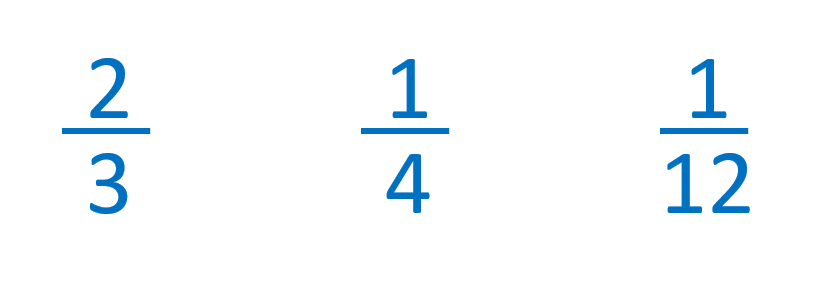The lowest common multiple of 3, 4 and 12 is 12. This means that 12 is the first number that can be divided exactly by 3, 4 and 12.

We will use 12 as our denominator.

We multiply both the numerators and denominators by the same value to write the fractions as equivalent fractions.We write   2 / 3   as   8 / 12   by multiplying the numerator and denominator by 4.

We write   1 / 4   as   3 / 12   by multiplying the numerator and denominator by 3.

1 / 12   is already out of 12, so we do not need to change it.Now that the fractions have the same denominator, we can write them as a ratio using their numerators.

We can write   8 / 12   ,   3 / 12   and   1 / 12   as 8 : 3 : 1.

We can check our answer by adding the values in the ratio.

8 + 3 + 1 = 12, which is our denominator on the bottom of the fractions.Now try our lesson on converting ratios to fractions where we learn how to write a ratio as a fraction.